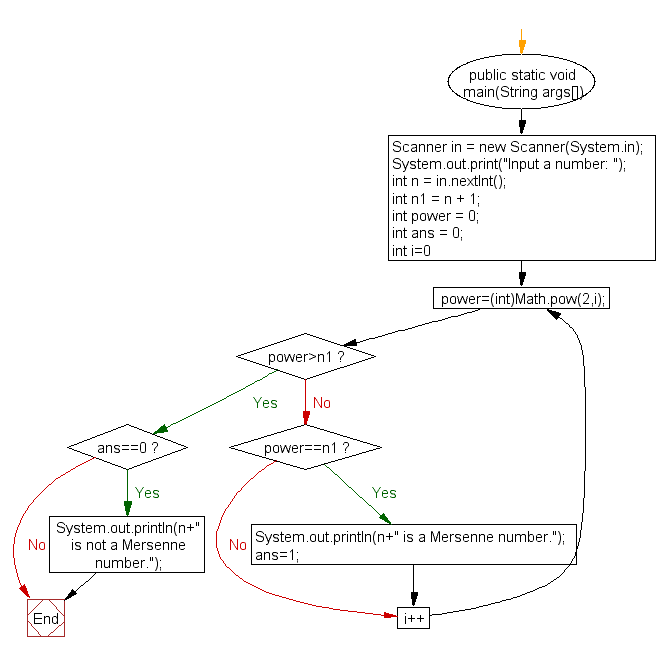﻿ Java exercises: Check if a number is Mersenne number or not - w3resource# Java Exercises: Check if a number is Mersenne number or not

## Java Numbers: Exercise-22 with Solution

Write a program to check if a number is Mersenne number or not.

In mathematics, a Mersenne number is a number that can be written in the form M(n) = 2n − 1 for some integer n.
The first four Mersenne primes are 3, 7, 31, and 127

Test Data
Input a number: 127

Pictorial Presentation:Sample Solution:

Java Code:

``````import java.util.Scanner;
import java.math.BigInteger;
public class Example22 {

public static void main(String args[])
{
Scanner in = new Scanner(System.in);
System.out.print("Input a number: ");
int n = in.nextInt();
int n1 = n + 1;

int power = 0;
int ans = 0;
for(int i=0;;i++)
{
power=(int)Math.pow(2,i);
if(power>n1)
{
break;
}
else if(power==n1)
{
System.out.println(n+" is a Mersenne number.");
ans=1;
}
}
if(ans==0)
{
System.out.println(n+" is not a Mersenne number.");
}
}
}
```
```

Sample Output:

```Input a number: 127
127 is a Mersenne number.
```

Flowchart:Java Code Editor:

What is the difficulty level of this exercise?

﻿

## Java: Tips of the Day

Array vs ArrayLists:

The main difference between these two is that an Array is of fixed size so once you have created an Array you cannot change it but the ArrayList is not of fixed size. You can create instances of ArrayLists without specifying its size. So if you create such instances of an ArrayList without specifying its size Java will create an instance of an ArrayList of default size.

Once an ArrayList is full it re-sizes itself. In fact, an ArrayList is internally supported by an array. So when an ArrayList is resized it will slow down its performance a bit as the contents of the old Array must be copied to a new Array.

At the same time, it's compulsory to specify the size of an Array directly or indirectly while creating it. And also Arrays can store both primitives and objects while ArrayLists only can store objects.

Ref: https://bit.ly/3o8L2KH